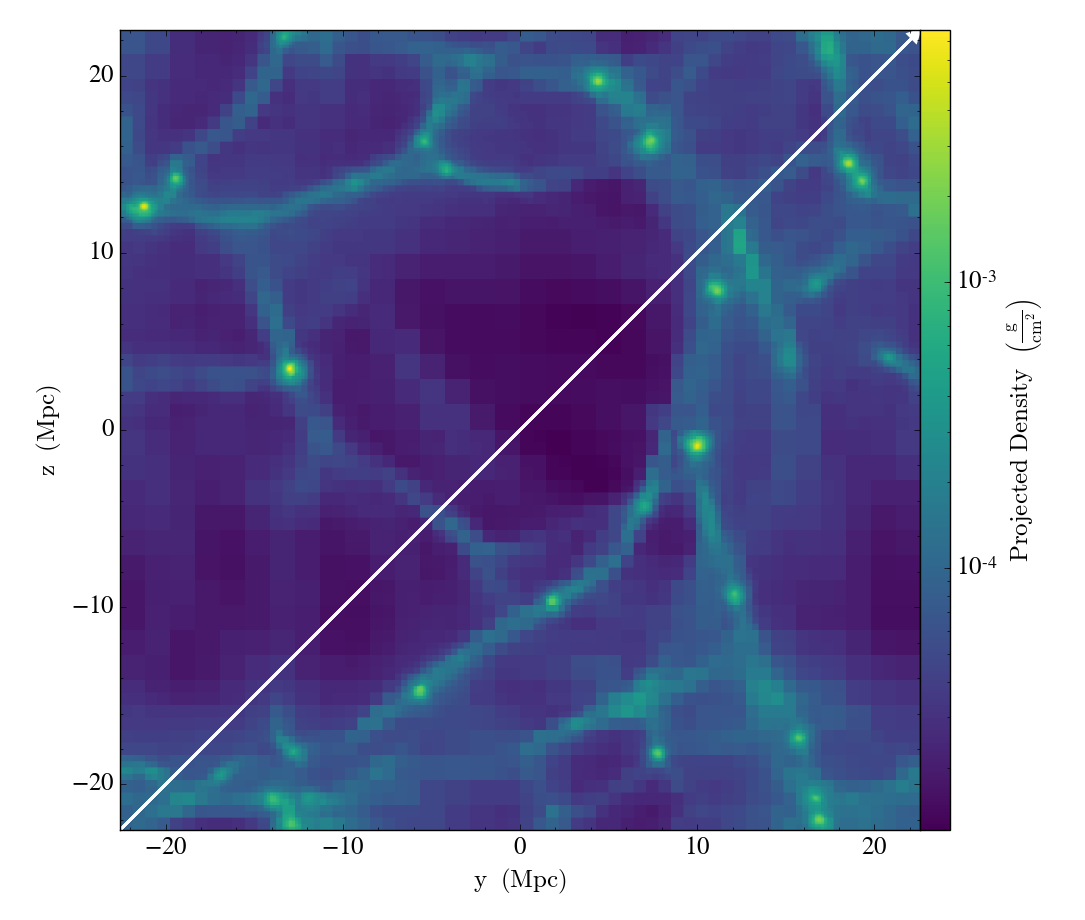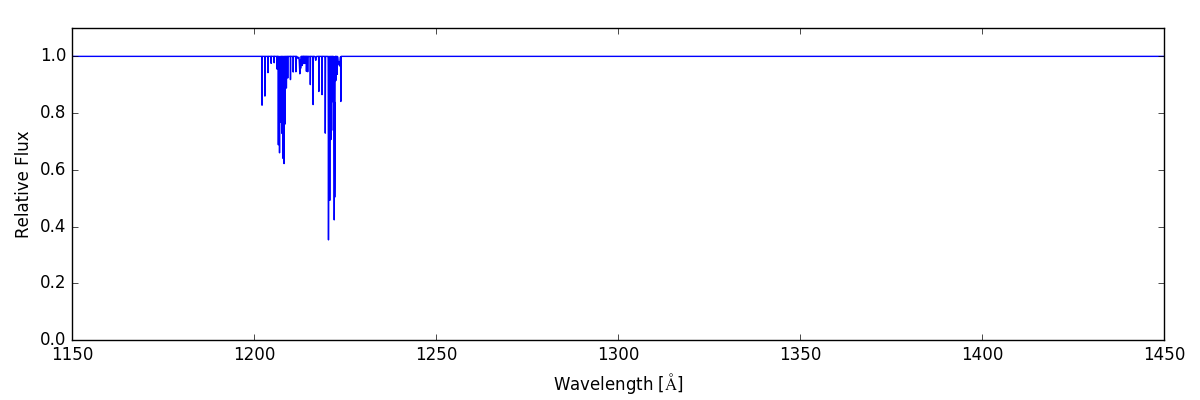# Annotated Example

The best way to get a feel for what Trident can do is to go through an annotated example of its use. This section will walk you through the steps necessary to produce a synthetic spectrum based on simulation data and to view its path through the parent dataset. The following example, available in the source code itself, can be applied to datasets from any of the different simulation codes that Trident and yt support, although it may require some tweaking of parameters for optimal performance. If you want to recreate the following analysis with the exact dataset used, it can be downloaded here.

The basic process for generating a spectrum and overplotting a sightline’s trajectory through the dataset goes in three steps:

1. Generate a `LightRay` from the simulation data representing a sightline through the data.

2. Define the desired spectrum features and use the `LightRay` to create a corresponding synthetic spectrum.

3. Create a projected image and overplot the path of the `LightRay`.

## Simple LightRay Generation

A `LightRay` is a 1D object representing the path a ray of light takes through a simulation volume on its way from some bright background object to the observer. It records all of the gas fields it intersects along the way for use in many tasks, including construction of a spectrum.

In order to generate a `LightRay` from your data, you need to first make sure that you’ve imported both the yt and Trident packages, and specify the filename of the dataset from which to extract the light ray:

```import yt
import trident
fn = 'enzo_cosmology_plus/RD0009/RD0009'
```

We need to decide the trajectory that the `LightRay` will take through our simulation volume. This arbitrary trajectory is specified with coordinates in code length units (e.g. [x_start, y_start, z_start] to [x_end, y_end, z_end]). Probably the simplest trajectory is cutting diagonally from the origin of the simulation volume to its outermost corner using the yt `domain_left_edge` and `domain_right_edge` attributes. Here we load the dataset into yt to get access to these attributes:

```ds = yt.load(fn)
ray_start = ds.domain_left_edge
ray_end = ds.domain_right_edge
```

Let’s define what lines or species we want to be added to our final spectrum. In this case, we want to deposit all hydrogen, carbon, nitrogen, oxygen, and magnesium lines to the resulting spectrum from the dataset:

```line_list = ['H', 'C', 'N', 'O', 'Mg']
```

We can now generate the light ray using the `make_simple_ray()` function by passing the dataset and the trajectory endpoints to it as well as telling trident to save the resulting ray dataset to an HDF5 file. We explicitly instruct trident to pull all necessary fields from the dataset in order to be able to add the lines from our `line_list`:

```ray = trident.make_simple_ray(ds,
start_position=ray_start,
end_position=ray_end,
data_filename="ray.h5",
lines=line_list)
```

The resulting `ray` is a `LightRay` object, consisting of a series of arrays representing the different fields it probes in the original dataset along its length. Each element in the arrays represents a different resolution element along the path of the ray. The ray also possesses some special fields not originally present in the original dataset:

• `('gas', l')` Location along the LightRay length from 0 to 1.

• `('gas', 'dl')` Pathlength of resolution element (not a true pathlength for particle-based codes)

• `('gas', 'redshift')` Cosmological redshift of resolution element

• `('gas', 'redshift_dopp')` Doppler redshift of resolution element

• `('gas', 'redshift_eff')` Effective redshift (combined cosmological and Doppler)

Like any dataset, you can see what fields are present on the ray by examining its `derived_field_list` (e.g., `print(ds.derived_field_list`). If you want more ions present on this ray than are currently shown, you can add them with `add_ion_fields` (see: Adding Ion Fields).

This `ray` object is also saved to disk as an HDF5 file, which can later be loaded into `yt` as a stand-alone dataset (e.g., `ds = yt.load('ray.h5')`).

## Overplotting a LightRay’s Trajectory on a Projection

Here we create a projection of the density field along the x axis of the dataset, and then overplot the path the `LightRay` takes through the simulation, before saving it to disk. The `annotate_ray()` operation should work for any volumentric plot, including slices, and off-axis plots:

```p = yt.ProjectionPlot(ds, 'x', 'density')
p.annotate_ray(ray, arrow=True)
p.save('projection.png')
```## Calculating Column Densities

Perhaps we wish to know the total column density of a particular ion present along this `LightRay`. This can easily be done by multiplying the desired ion number density field by the pathlength field, `dl`, to yield an array of column densities for each resolution element, and then summing them together:

```column_density_HI = ray.r[('gas', 'H_p0_number_density')] * ray.r[('gas', 'dl')]
print('HI Column Density = %g' % column_density_HI.sum())
```

## Spectrum Generation

Now that we have our `LightRay` we can use it to generate a spectrum. To create a spectrum, we need to make a `SpectrumGenerator` object defining our desired wavelength range and bin size. You can do this by manually setting these features, or just using one of the presets for an instrument. Currently, we have three pre-defined instruments, the G130M, G160M, and G140L observing modes for the Cosmic Origins Spectrograph aboard the Hubble Space Telescope: `COS-G130M`, `COS-G160M`, and `COS-G140L`. Notably, instrument `COS` aliases to `COS-G130M`.

We then use this `SpectrumGenerator` object to make a raw spectrum according to the intersecting fields it encountered in the corresponding `LightRay`. We save this spectrum to disk, and plot it:

```sg = trident.SpectrumGenerator('COS-G130M')
sg.make_spectrum(ray, lines=line_list)
sg.save_spectrum('spec_raw.txt')
sg.plot_spectrum('spec_raw.png')
```From here we can do some post-processing to the spectrum to include additional features that would be present in an actual observed spectrum. We add a background quasar spectrum, a Milky Way foreground, apply the COS line spread function, and add gaussian noise with SNR=30:

```sg.add_qso_spectrum()
sg.apply_lsf()
```

Finally, we use plot and save the resulting spectrum to disk:

```sg.save_spectrum('spec_final.txt')
sg.plot_spectrum('spec_final.png')
```

which produces:To create more complex or ion-specific spectra, refer to Advanced Spectrum Generation.

## Compound LightRays

In some cases (e.g. studying redshift evolution of the IGM), it may be desirable to create a `LightRay` that covers a range in redshift that is larger than the domain width of a single simulation snaptshot. Rather than simply sampling the same dataset repeatedly, which is inherently unphysical since large scale structure evolves with cosmic time, Trident allows the user to create a ray that samples multiple datasets from different redshifts to produce a much longer ray that is continuous in redshift space. This is done by using the `make_compound_ray()` function. This function is similar to the previously mentioned `make_simple_ray()` function, but instead of accepting an individual dataset, it takes a simulation parameter file, the associated simulation type, and the desired range in redshift to be probed by the ray, while still allowing the user to specify the same sort of line list as before::

```fn = 'enzo_cosmology_plus/AMRCosmology.enzo'
ray = trident.make_compound_ray(fn, simulation_type='Enzo',
near_redshift=0.0, far_redshift=0.1,
lines=line_list)
```

In this example, we’ve created a ray from an Enzo simulation (the same one used above) that goes from z = 0 to z = 0.1. This ray can now be used to generate spectra in the exact same ways as before.

Obviously, there need to be sufficient simulation outputs over the desired redshift range of the compound ray in order to have continuous sampling. To assure adequate simulation output frequency for this, one can use yt’s `plan_cosmology_splice()` function. See an example of its usage in the yt_astro_analysis documentation.

We encourage you to look at the detailed documentation for `make_compound_ray()` in the API Reference section to understand how to control how the ray itself is constructed from the available data.

Note